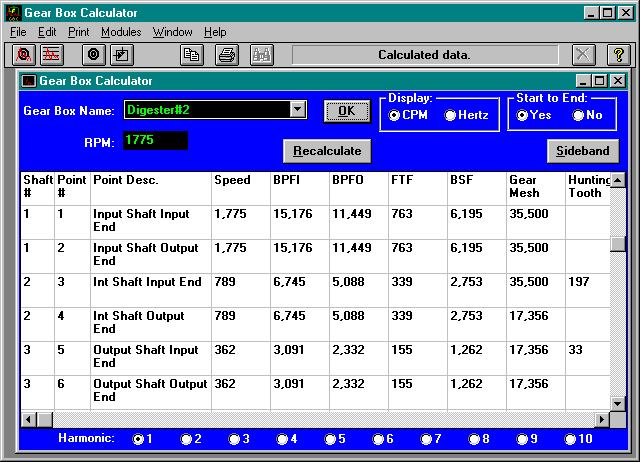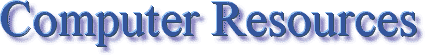## Bearing Data Module

The Bearing Data Module contains the essential bearing data to calculate bearing frequencies.

Over 21,000 bearings are already included in the database and many more can be added. For reference and cross reference the bearing I.D. and O.D. as well as the AFBMA number can be included.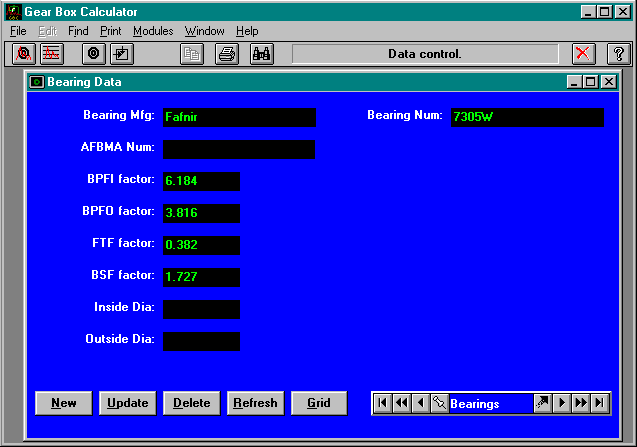## Gear Box Data Module

The Gear Box Data Module contains the elements necessary to build gear boxes. Gear boxes are made up from:

• Bearing
• Shafts
• Gears

The idea behind building a database of gearboxes is that once the gear box has been defined and saved, calculating gear box frequencies consists of nothing more than clicking a button.

Click here to view a schematic of a gearbox that is used in the Gear Box Calculator tutorial. You will notice that the gear box is made up of a number of different elements such as a bearings, shafts, and gears.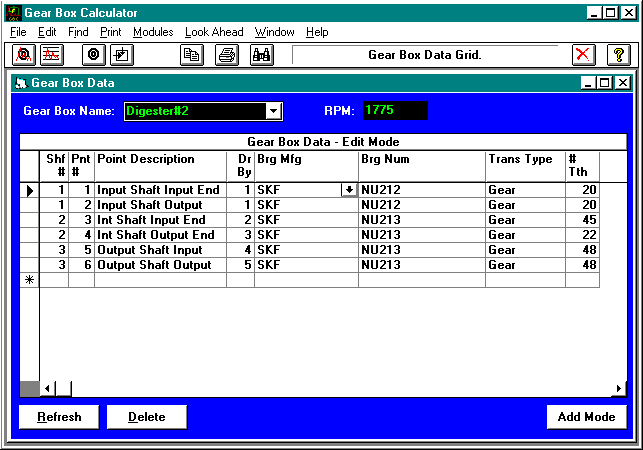## Bearing Calculator Module

The Bearing Calculator Module calculates bearing frequencies for any bearing in the database.

A new feature to this program is the ability to calculate sideband frequencies off of any of the frequencies calculated by the Bearing Calculator.

Frequencies can be calculated off of:

• BPFI - Ball Pass Frequency Inner Race
• BPFO - Ball Pass Frequency Outer Race
• BSF - Ball Spin Frequency
• FTF - Fundamental Train Frequency

You supply the RPM of the bearing and the program does the rest. The screen also displays those calculations for 10 harmonics and speeds.

10 harmonics for all frequencies can also be calculated by clicking the correct Harmonic radio button.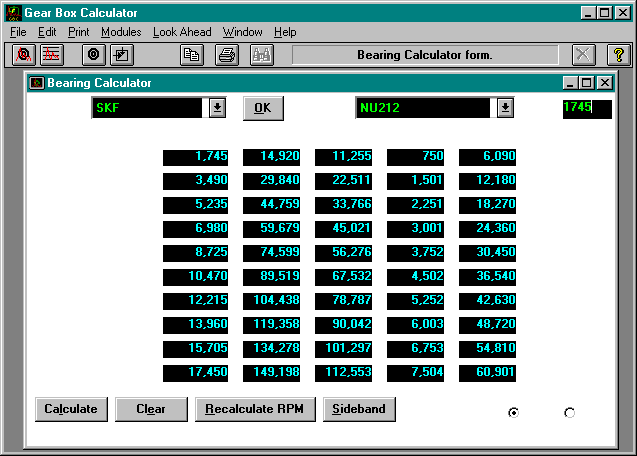## Gear Box Calculator Module

The Gear Box Calculator Module calculates vibration frequencies for an entire gear box. All of the speed differences because of gears are taken into account automatically.

Two new features of this program are the abilities to calculate Hunting Tooth frequencies and to calculate Sideband frequencies off of any of the frequencies generated by the Gear Box Calculator.

Frequencies can be calculated off of:

• A starting RPM
• An ending RPM

10 harmonics for all frequencies can also be calculated by clicking the correct Harmonic radio button.